# Question 2 1 pts Which statement(s) concerning the reaction is/are true? 2ABig) Alg) + Bale) K-2.9...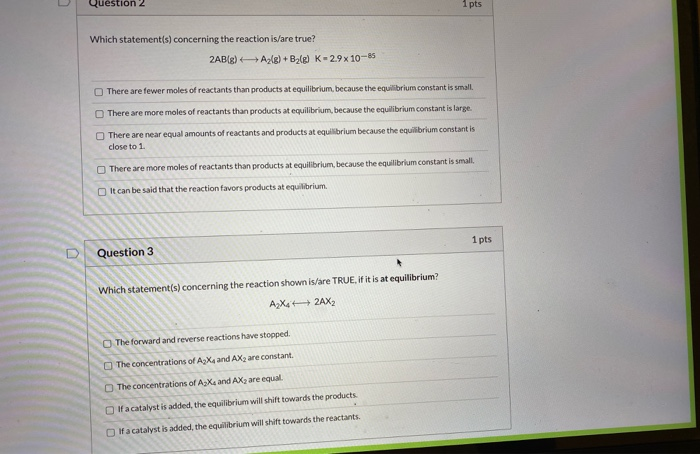Question 2 1 pts Which statement(s) concerning the reaction is/are true? 2ABig) Alg) + Bale) K-2.9 x 10-85 There are fewer moles of reactants than products at equilibrium, because the equilibrium constant is small There are more moles of reactants than products at equilibrium, because the equilibrium constant is large. There are near equal amounts of reactants and products at equilibrium because the equilibrium constant is close to 1 There are more moles of reactants than products at equilibrium, because the equilibrium constant is small, It can be said that the reaction favors products at equilibrium. 1 pts Question 3 Which statement(s) concerning the reaction shown is/are TRUE, if it is at equilibrium? AX: 42AX2 The forward and reverse reactions have stopped. The concentrations of AX and AX2 are constant The concentrations of AX and AX2 are equal. If a catalyst is added, the equilibrium will shift towards the products. If a catalyst is added, the equilibrium will shift towards the reactants.

Q.2) The correct statement is (d) There are more moles of reactants than product at equilibrium because the equilibrium constant is small.

Q.3) The correct statements are (b) The concentration of A2X2 and AX2 are constant at equilibrium.

(d)If a catalyst is added, the equilibrium is shift towards product.

##### Add Answer of: Question 2 1 pts Which statement(s) concerning the reaction is/are true? 2ABig) Alg) + Bale) K-2.9...
Similar Homework Help Questions
• ### Question 3 1 pts Which statement(s) concerning the reaction shown is/are TRUE, if it is at...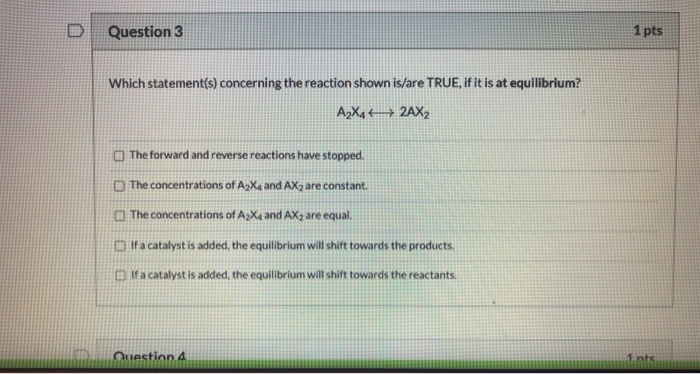Question 3 1 pts Which statement(s) concerning the reaction shown is/are TRUE, if it is at equilibrium? A2X442AX2 The forward and reverse reactions have stopped. The concentrations of AX and AX, are constant. The concentrations of AX and AX2 are equal. If a catalyst is added, the equilibrium will shift towards the products. If a catalyst is added, the equilibrium will shift towards the reactants. Question 4 1. nts

• ### D Question 2 1 pts Which statement(s) concerning the reaction is/are true? 2A) A2(g) +B2(g) K-29x...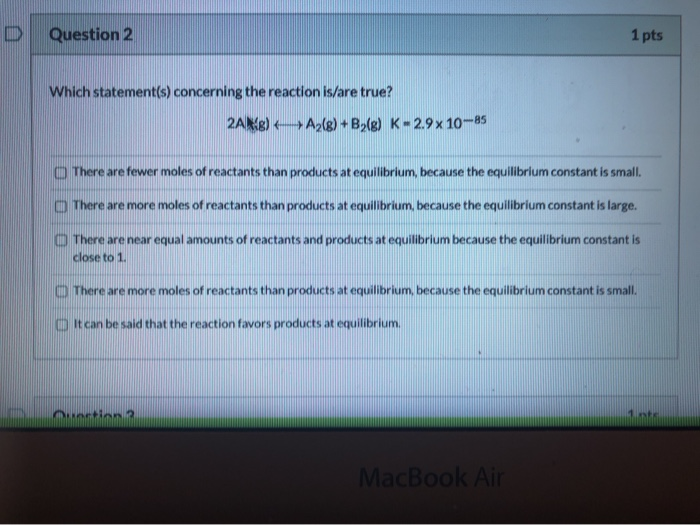D Question 2 1 pts Which statement(s) concerning the reaction is/are true? 2A) A2(g) +B2(g) K-29x 10-85 There are fewer moles of reactants than products at equilibrium, because the equilibrium constant is small. There are more moles of reactants than products at equilibrium, because the equilibrium constant is large. There are near equal amounts of reactants and products at equilibrium because the equilibrium constant is close to 1 There are more moles of reactants than products at equilibrium, because the...

• ### thanks for the help in advance! es/157542/quizzes/secured#lockdown Choose the statement below that is TRUE given the...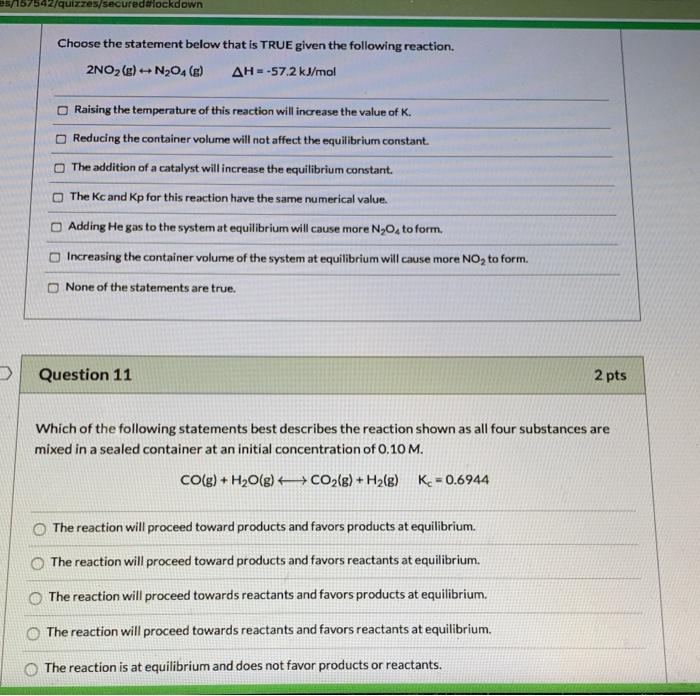thanks for the help in advance! es/157542/quizzes/secured#lockdown Choose the statement below that is TRUE given the following reaction. 2NO2(g) + N204 () AH-57.2 kJ/mol Raising the temperature of this reaction will increase the value of K. Reducing the container volume will not affect the equilibrium constant. The addition of a catalyst will increase the equilibrium constant. The Kc and Kp for this reaction have the same numerical value. Adding He gas to the system at equilibrium will cause more N30,...

• ### D Question 18 1 pts Which of the following statements best describes the reaction shown as...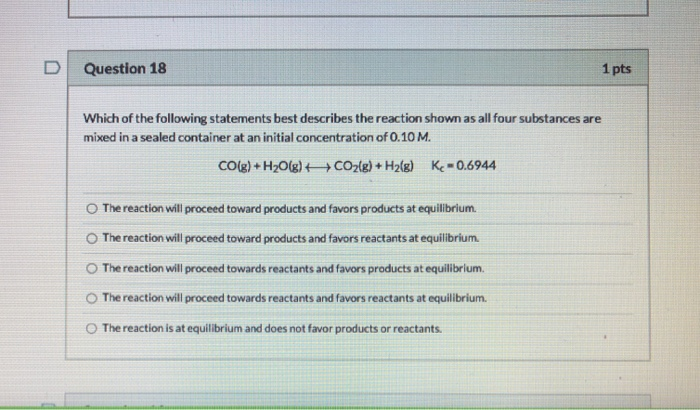D Question 18 1 pts Which of the following statements best describes the reaction shown as all four substances are mixed in a sealed container at an initial concentration of 0.10 M. CO(g) + H2O(g) + CO2(g) + H2(g) K-0.6944 The reaction will proceed toward products and favors products at equilibrium. The reaction will proceed toward products and favors reactants at equilibrium The reaction will proceed towards reactants and favors products at equilibrium. The reaction will proceed towards reactants and...

• ### Question 18 1 pts Which of the following statements best describes the reaction shown as all...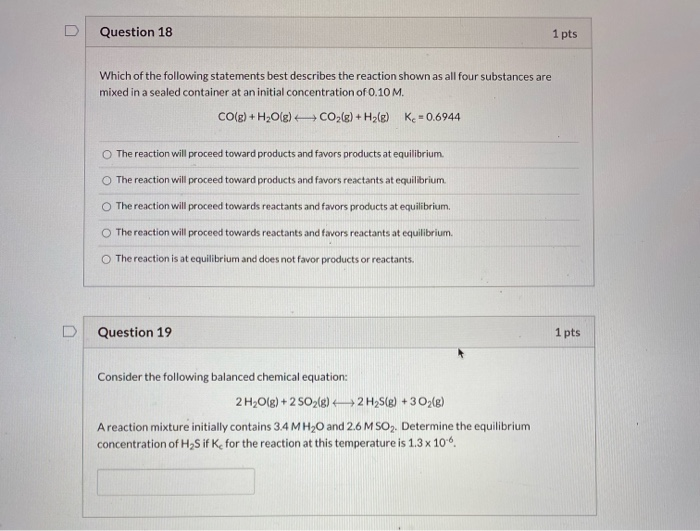Question 18 1 pts Which of the following statements best describes the reaction shown as all four substances are mixed in a sealed container at an initial concentration of 0.10 M. CO(g) + H2O(g) + CO2(g) + H2(E) K-0.6944 The reaction will proceed toward products and favors products at equilibrium. The reaction will proceed toward products and favors reactants at equilibrium The reaction will proceed towards reactants and favors products at equilibrium. The reaction will proceed towards reactants and favors...

• ### 48. If the conversion of A to B is slow and B to C is fast, what is the rate equation for this reaction? A) Rate =...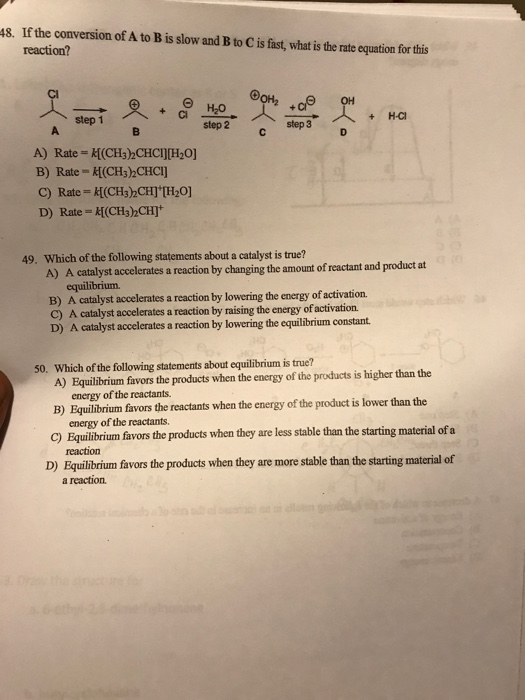48. If the conversion of A to B is slow and B to C is fast, what is the rate equation for this reaction? A) Rate = K[(CH3CHCI][H20] B) Rate - K[(CH3)2CHCI] C) Rate = K[(CH3)CH] [H0] D) Rate = {[(CH3)2CH] 49. Which of the following statements about a catalyst is true? A) A catalyst accelerates a reaction by changing the amount of reactant and product at equilibrium B) A catalyst accelerates a reaction by lowering the energy of activation...

• ### Check all of the following that are true concerning a reaction that is not extensive.

Check all of the following that are true concerning a reaction that is not extensive.Starting with equal amounts of reactants and products, the rate of the forward reaction is faster than the rate of the reverse reaction.The value of ?G^o is positive.At equilibrium, there will be mostly products.None of these statements is true.Starting with equal amounts of reactants and products, the forward reaction is spontaneous.

• ### QUESTION 5 Which of the following statements about a catalyst is true? Catalysts decrease the activation...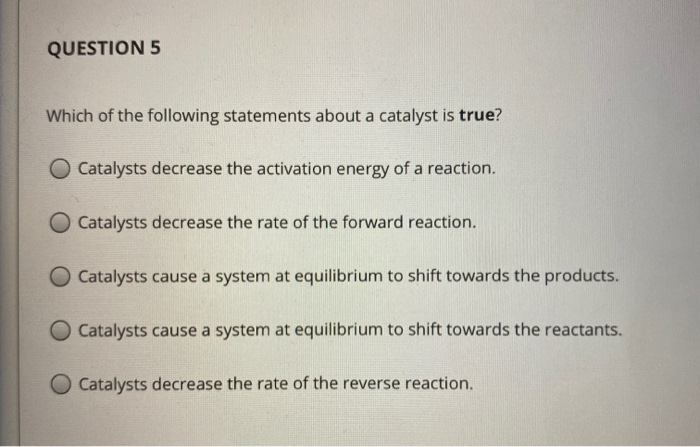QUESTION 5 Which of the following statements about a catalyst is true? Catalysts decrease the activation energy of a reaction. Catalysts decrease the rate of the forward reaction. Catalysts cause a system at equilibrium to shift towards the products. Catalysts cause a system at equilibrium to shift towards the reactants. Catalysts decrease the rate of the reverse reaction.

• ### QUESTION 5 Which of the following statements about a catalyst is true? O Catalysts increase the...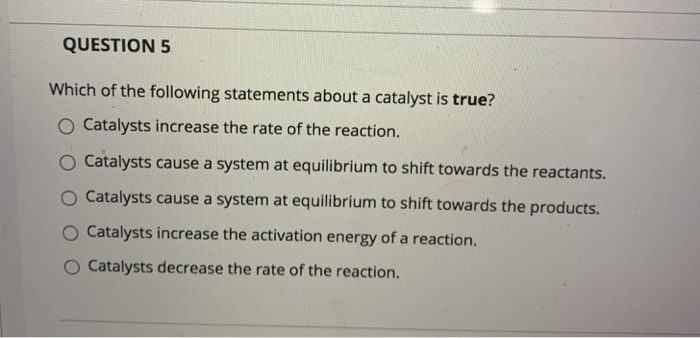QUESTION 5 Which of the following statements about a catalyst is true? O Catalysts increase the rate of the reaction. Catalysts cause a system at equilibrium to shift towards the reactants. o Catalysts cause a system at equilibrium to shift towards the products. Catalysts increase the activation energy of a reaction. Catalysts decrease the rate of the reaction. Show transcribed image text

• ### Which of the following is a true statement about chemical equilibria in general? equilibrium [products] = [reactants].

Which of the following is a true statement about chemical equilibria in general? A. At equilibrium the total concentration of products equals the total concentration of reactants, that is, [products] = [reactants]. B. Equilibrium is the result of the cessation of all chemical change. C. There is only one set of equilibrium concentrations that equals the Kc value. D. At equilibrium, the rate constant of the forward reaction is equal to the rate constant for the reverse reaction. E. At...

Free Homework App